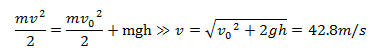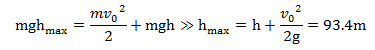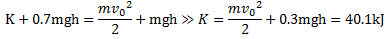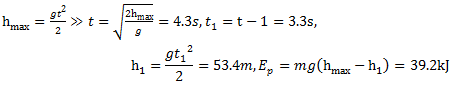# Answer to Question #5326 in Mechanics | Relativity for sharif ibrahim

Question #5326
A 100g stone is thrown straight upwards at 19 m/s from the top of a 75 m building.

(a) What is the Stone's velocity as it hits the ground?
(b) What is the maximum height of the stone?
(c)What is the stone's kinetic energy when the gravitational potential energy is 30% less than when it started?
(d) What is the stone's gravitational potential energy 1.0 s before it touches the ground?
1
2011-11-29T09:03:44-0500
m=100 kg
h=75m
v0=19m/s
a) When the stone hits the ground its the gravitational potential energy is equal zero, so lets use the law of energy conservation:b) The maximum height of the stone is achieved when the stones kinetic energy is equal zero. So lets use the law of energy conservation:c) Let`s use the law of energy conservation:d) We need use the time of the falla) 42.8 m/s
b) 93.4 m
c) 40.1 kj
d) 39.2 kj

Need a fast expert's response?

Submit order

and get a quick answer at the best price

for any assignment or question with DETAILED EXPLANATIONS!